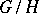# Matsushima criterion

(diff) ← Older revision | Latest revision (diff) | Newer revision → (diff)
The homogeneous space, whereis an affine reductive algebraic group (cf. also Affine group; Reductive group) defined over an algebraically closed fieldandis a closed subgroup of, is an affine algebraic variety if and only ifis a reductive group. This criterion was first found by Y. Matsushima  in the case whereis the complex field. Later, proofs were given that are valid for every algebraically closed field of characteristic zero (see , , ). In the case where the characteristic ofis positive, the proof of the criterion was obtained only after the proof of the Mumford hypothesis (see , ).